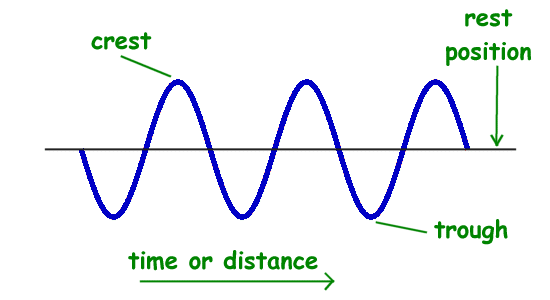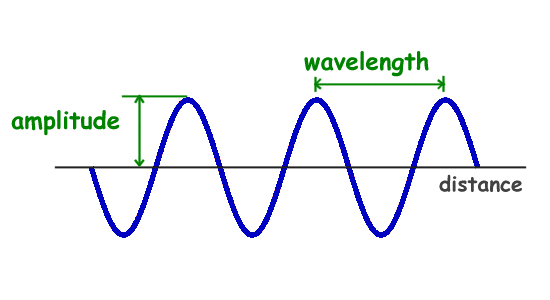Parents and Teachers: Support Ducksters by following us onor.

Science >> Physics for Kids

# Physics for Kids

## Properties of Waves

There are many properties that scientists use to describe waves. They include amplitude, frequency, period, wavelength, speed, and phase. Each of these properties is described in more detail below.

Graphing a Wave

When drawing a wave or looking at a wave on a graph, we draw the wave as a snapshot in time. The vertical axis is the amplitude of the wave while the horizontal axis can be either distance or time.In this picture you can see that the highest point on the graph of the wave is called the crest and the lowest point is called the trough. The line through the center of the wave is the resting position of the medium if there was no wave passing through.

We can determine a number of wave properties from the graph.

Amplitude

The amplitude of a wave is a measure of the displacement of the wave from its rest position. The amplitude is shown on the graph below.Amplitude is generally calculated by looking on a graph of a wave and measuring the height of the wave from the resting position.

The amplitude is a measure of the strength or intensity of the wave. For example, when looking at a sound wave, the amplitude will measure the loudness of the sound. The energy of the wave also varies in direct proportion to the amplitude of the wave.

Wavelength

The wavelength of a wave is the distance between two corresponding points on back-to-back cycles of a wave. This can be measured between two crests of a wave or two troughs of a wave. The wavelength is usually represented in physics by the Greek letter lambda (λ).

Frequency and Period

The frequency of a wave is the number of times per second that the wave cycles. Frequency is measured in Hertz or cycles per second. The frequency is often represented by the lower case "f."

The period of the wave is the time between wave crests. The period is measured in time units such as seconds. The period is usually represented by the upper case "T."

The period and frequency are closely related to each other. The period equals 1 over the frequency and the frequency is equal to one over the period. They are reciprocals of each other as shown in the following formulas.

period = 1/frequency
or
T = 1/f

frequency = 1/period
or
f = 1/T

Speed or Velocity of a Wave

Another important property of a wave is the speed of propagation. This is how fast the disturbance of the wave is moving. The speed of mechanical waves depends on the medium that the wave is traveling through. For example, sound will travel at a different speed in water than in air.

The velocity of a wave is usually represented by the letter "v." The velocity can be calculated by multiplying the frequency by the wavelength.

velocity = frequency * wavelength
or
v = f * λ

Activities

Science >> Physics for KidsFollow us onor